# Cache-Oblivious Data Structures:Fundamental Primitives

Fundamental Primitives

The most fundamental cache-oblivious primitive is scanning—scanning an array with N elements incurs Θ( N ) memory transfers for any value of B. Thus algorithms such as median ﬁnding and data structures such as stacks and queues that only rely on scanning are automatically cache-oblivious. In fact, the examples above are optimal in the cache-oblivious model. Other examples of algorithms that only rely on scanning include Quicksort and Mergesort. However, they are not asymptotically optimal in the cache-oblivious model since they use O( N log N ) memory transfers rather than Θ(Sort M,B (N )).

Apart from algorithms and data structures that only utilize scanning, most cache-oblivious results use recursion to obtain eﬃciency; in almost all cases, the sizes of the recursive problems decrease double-exponentially. In this section we describe two of the most fundamental such recursive schemes, namely the van Emde Boas layout and the k-merger.

Van Emde Boas Layout

One of the most fundamental data structures in the I/O-model is the B-tree . A B-tree is basically a fanout Θ(B) tree with all leaves on the same level. Since it has height O(logB N ) and each node can be accessed in O(1) memory transfers, it supports searches in O(logB N ) memory transfers. It also supports range queries, that is, the reporting of all K elements in a given query range, in O(logB N + K ) memory transfers. Since B is an integral part of the deﬁnition of the structure, it seems challenging to develop a cache-oblivious B-tree structure. However, Prokop  showed how a binary tree can be laid out in memory in order to obtain a (static) cache-oblivious version of a B-tree. The main idea is to use a recursively deﬁned layout called the van Emde Boas layout closely related to the deﬁnition of a van Emde Boas tree . The layout has been used as a basic building block of most cache-oblivious search structures (e.g in [1, 8, 10–12, 18, 25]).

Layout

For simplicity, we only consider complete binary trees. A binary tree is complete if it has N = 2h 1 nodes and height h for some integer h. The basic idea in the van Emde Boas layout of a complete binary tree T with N leaves is to divide T at the middle level and lay out the pieces recursively (Figure 34.3). More precisely, if T only has one node it is simply laid out as a single node in memory. Otherwise, we deﬁne the top tree T0 to be the subtree consisting of the nodes in the topmost lh/2J levels of T , and the bottom trees T1,…, Tk to be the Θ() subtrees rooted in the nodes on level lh/2l of T ; note that all the subtrees have size Θ(). The van Emde Boas layout of T consists of the van Emde Boas layout of T0 followed by the van Emde Boas layouts of T1,... , Tk .

Search

To analyze the number of memory transfers needed to perform a search in T , that is, traverse a root-leaf path, we consider the ﬁrst recursive level of the van Emde Boas layout where the subtrees are smaller than B. As this level T is divided into a set of base trees of size between Θ() and Θ(B), that is, of height Ω(log B) (Figure 34.4). By the deﬁnition of the layout, each base tree is stored in O(B) contiguous memory locations and can thus be accessed in O(1) memory transfers. That the search is performed in O(logB N ) memory transfers then follows since the search path traverses O((log N )/ log B) = O(logB N ) diﬀerent base trees.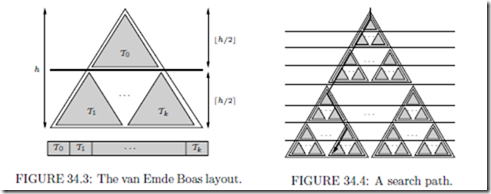Range query

To analyze the number of memory transfers needed to answer a range query [x1, x2] on T using the standard recursive algorithm that traverses the relevant parts of T (starting at the root), we ﬁrst note that the two paths to x1 and x2 are traversed in O(logB N ) memory transfers. Next we consider traversed nodes v that are not on the two paths to x1 and x2. Since all elements in the subtree Tv rooted at such a node v are reported, and since a subtree of height log B stores Θ(B) elements, O( K ) subtrees Tv of height log B are visited. This in turn means that the number of visited nodes above the last log B levels of T is also O( K ); thus they can all be accessed in O( K ) memory transfers. Consider the smallest recursive level of the van Emde Boas layout that completely contain Tv . This level is of size between Ω(B) and O(B2 ) (Figure 34.5(a)). On the next level of recursion Tv is broken into a top part and O(B) bottom parts of size between B) and O(B) each (Figure 34.5(b)). The top part is contained in a recursive level of size O(B) and is thus stored within O(B) consecutive memory locations; therefore it can be accessed in O(1) memory accesses. Similarly, the O(B) nodes in the O(B) bottom parts are stored consecutively in memory; therefore they can all be accessed in a total of O(1) memory transfers. Therefore, the optimal paging strategy can ensure that any traversal of Tv is performed in O(1) memory transfers, simply by accessing the relevant O(1) blocks. Thus overall a range query is performed in O(logB N + K ) memory transfers.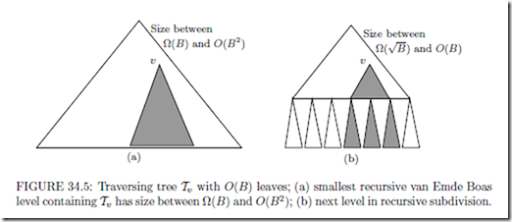THEOREM 34.1 Let T be a complete binary tree with N leaves laid out using the van Emde Boas layout. The number of memory transfers needed to perform a search (traverse a root-to-leaf path) and a range query in T is O(logB N ) and O(logB N + K ), respectively.

Note that the navigation from node to node in the van Emde Boas layout is straight- forward if the tree is implemented using pointers. However, navigation using arithmetic on array indexes is also possible . This avoids the use of pointers and hence saves space.

The constant in the O(logB N ) bound for searching in Theorem 34.1 can be seen to be four. Further investigations of which constants are possible for cache-oblivious comparison based searching appear in .

k-Merger

In the I/O-model the two basic optimal sorting algorithms are multi-way versions of Merge- sort and distribution sorting (Quicksort) . Similarly, Frigo et al.  showed how both merge based and distribution based optimal cache-oblivious sorting algorithms can be developed. The merging based algorithm, Funnelsort, is based on a so-called k-merger. This structure has been used as a basic building block in several cache-oblivious algorithms. Here we describe a simpliﬁed version of the k-merger due to Brodal and Fagerberg .

Binary mergers and merge trees

A binary merger merges two sorted input streams into a sorted output stream: In one merge step an element is moved from the head of one of the input streams to the tail of the output stream; the heads of the input streams, as well as the tail of the output stream, reside in buﬀers of a limited capacity.

Binary mergers can be combined to form binary merge trees by letting the output buﬀer of one merger be the input buﬀer of another—in other words, a binary merge tree is a binary tree with mergers at the nodes and buﬀers at the edges, and it is used to merge a set of sorted input streams (at the leaves) into one sorted output stream (at the root). Refer to Figure 34.6 for an example.

An invocation of a binary merger in a binary merge tree is a recursive procedure that performs merge steps until the output buﬀer is full (or both input streams are exhausted); if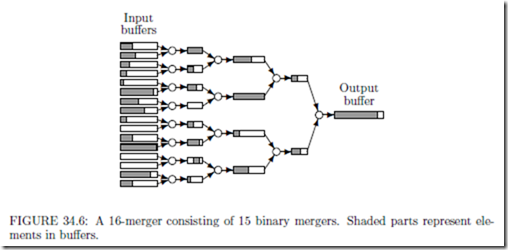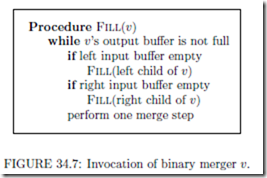an input buﬀer becomes empty during the invocation (and the corresponding stream is not exhausted), the input buﬀer is recursively ﬁlled by an invocation of the merger having this buﬀer as output buﬀer. If both input streams of a merger get exhausted, the corresponding output stream is marked as exhausted. A procedure Fill(v) performing an invocation of a binary merger v is shown in Figure 34.7 (ignoring exhaustion issues). A single invocation Fill(r) on the root r of a merge tree will merge the streams at the leaves of the tree.

kmerger

A k-merger is a binary merge tree with speciﬁc buﬀer sizes. For simplicity, we assume that k is a power of two, in which case a k-merger is a complete binary tree of k 1 binary mergers. The output buﬀer at the root has size k3, and the sizes of the rest of the buﬀers are deﬁned recursively in a manner resembling the deﬁnition of the van Emde Boas layout: Let i = log k be the height of the k-merger. We deﬁne the top tree to be the subtree consisting of all mergers of depth at most li/2l, and the bottom trees to be the subtrees rooted in nodes at depth li/2l + 1. We let the edges between the top and bottom trees have buﬀers of size k3/2, and deﬁne the sizes of the remaining buﬀers by recursion on the top and bottom trees. The input buﬀers at the leaves hold the input streams and are not part of the k-merger deﬁnition. The space required by a k-merger, excluding the output buﬀer at the root, is given by S(k) = k1/2 · k3/2 + (k1/2 + 1) · S(k1/2), which has the solution S(k) = Θ(k2).

We now analyze the number of memory transfers needed to ﬁll the output buﬀer of size k3 at the root of a k-merger. In the recursive deﬁnition of the buﬀer sizes in the k-merger, consider the ﬁrst level where the subtrees (excluding output buﬀers) have size less than M/3; if k¯ is the number of leaves of one such subtree, we by the space usage of k-mergers have k¯2 M/3 and (k¯2)2 = k¯4 = Ω(M ). We call these subtrees of the k-merger base trees and the buﬀers between the base trees large buﬀers. Assuming B2 M/3, a base tree Tv rooted in v together with one block from each of the large buﬀers surrounding it (i.e., its single output buﬀer and k¯ input buﬀers) can be contained in fast memory, since M/3+ B + k¯ · B M/3+ B + (M/3)1/2 · (M/3)1/2 M . If the k-merger consists of a single base tree, the number of memory transfers used to ﬁll its output buﬀer with k3 elements during an invocation is trivially O(k3/B + k). Otherwise, consider an invocation of the root v of a base tree Tv , which will ﬁll up the size Ω(k¯3) output buﬀer of v. Loading Tv and one block for each of the k¯ buﬀers just below it into fast memory will incur O(k¯2/B + k¯) memory transfers. This is O(1/B) memory transfer for each of the Ω(k¯3) elements output, since k¯4 = Ω(M ) implies k¯2 = Ω(M 1/2) = Ω(B), from which k¯ = O(k¯3/B) follows. Provided  that none of the input buﬀers just below Tv become empty, the output buﬀer can then be ﬁlled in O(k¯3/B) memory transfers since elements can be read from the input buﬀers in O(1/B) transfers amortized. If a buﬀer below Tv becomes empty, a recursive invocation is needed. This invocation may evict Tv from memory, leading to its reloading when the invocation ﬁnishes. We charge this cost to the Ω(k¯3) elements in the ﬁlled buﬀer, or O(1/B)

memory transfers per element. Finally, the last time an invocation is used to ﬁll a particular buﬀer, the buﬀer may not be completely ﬁlled (due to exhaustion). However, this happens only once for each buﬀer, so we can pay the cost by charging O(1/B) memory transfers to each position in each buﬀer in the k-merger. As the entire k-merger uses O(k2) space and merges k3 elements, these charges add up to O(1/B) memory transfers per element.

We charge an element O(1/B) memory transfers each time it is inserted into a large buﬀer. Since k¯ = Ω(M 1/4), each element is inserted in O(logk¯ k) = O(logM k3) large buﬀers. Thus we have the following.

THEOREM 34.2 Excluding the output buﬀers, the size of a k-merger is O(k2) and it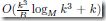memory transfers during an invocation to ﬁll up its output buﬀer of size k3.

Funnelsort

The cache-oblivious sorting algorithm Funnelsort is easily obtained once the k-merger structure is deﬁned: Funnelsort breaks the N input elements into N 1/3 groups of size N 2/3, sorts them recursively, and then merges the sorted groups using an N 1/3-merger.

Funnelsort can be analyzed as follows: Since the space usage of a k-merger is sub-linear in its output, the elements in a recursive sort of size M/3 only need to be loaded into memory once during the entire following recursive sort. For k-mergers at the remaining higher levels in the recursion tree, we have k3 M/3 B2, which implies k2 B4/3 > B and hence k3/B > k. By Theorem 34.2, the number of memory transfers during a merge involving N ! elements is then O(logM (N !)/B) per element. Hence, the total number of memory transfers per element is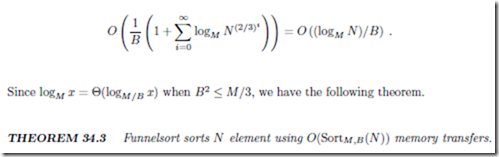In the above analysis, the exact (tall cache) assumption on the size of the fast memory is B2 M/3. In  it is shown how to generalize Funnelsort such that it works un- der the weaker assumption B1+ε M , for ﬁxed ε > 0. The resulting algorithm incurs the optimal O(SortM,B (N )) memory transfers when B1+ε = M , at the price of incurring O( 1 · SortM,B (N )) memory transfers when B2 M . It is shown in  that this trade-oﬀ is the best possible for comparison based cache-oblivious sorting.